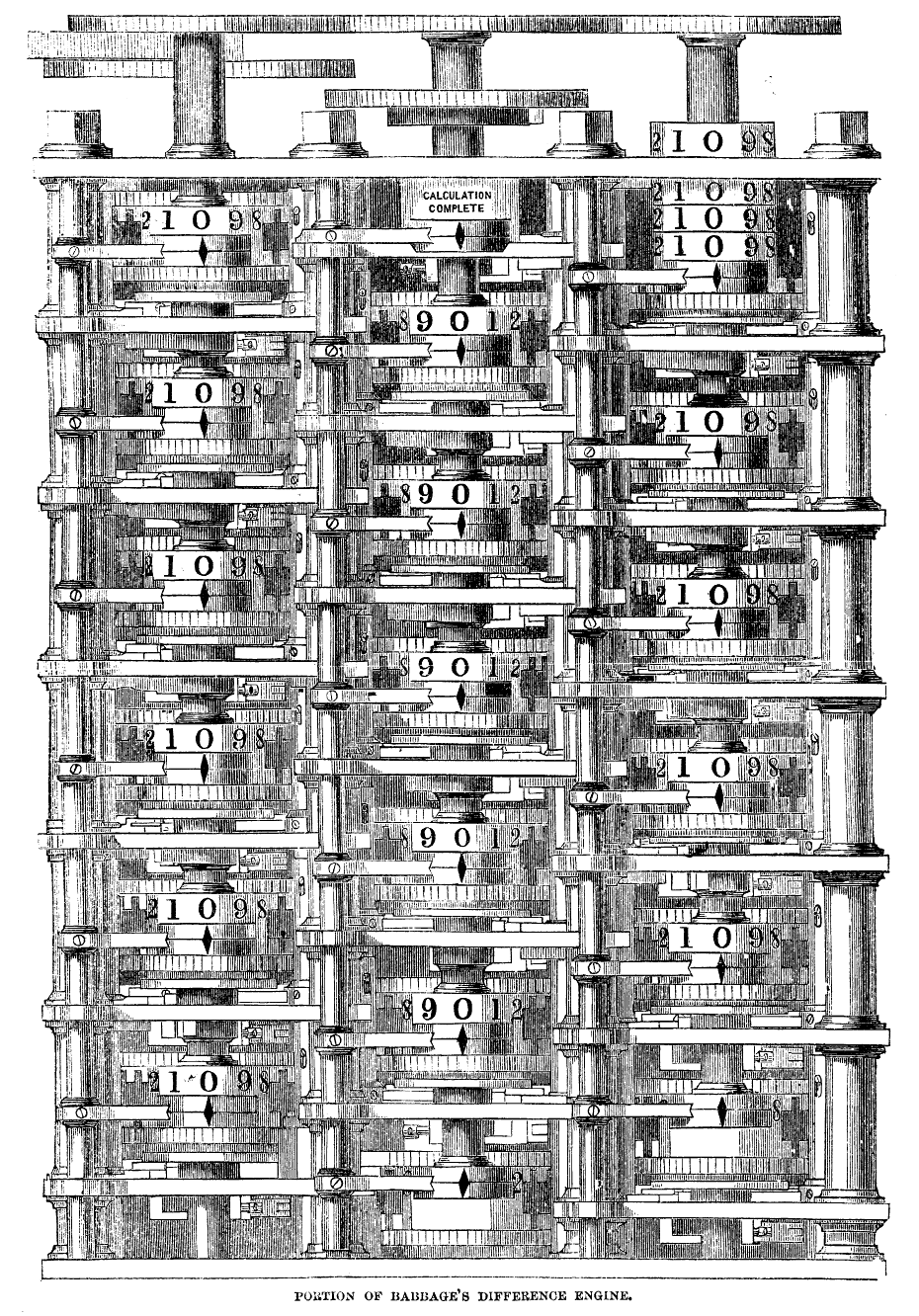October 18th, 2021 marks the 150th anniversary of the death of polymath Charles Babbage, who conceived of many of the ideas behind the modern computer in the early 1800s. His mechanical "difference engine" — powered by turning a crank handle — could quickly compute the value of polynomials, and as such could help with the computational steps of problems like this one:

Consider the quadratic + n + 41. Let's try some nonnegative integer values of n and see what we get:

\begin{align*} n=0 \rightarrow\quad& 0^2 + 0 + 41 = 41 \quad\text{prime!}\\ n=1 \rightarrow\quad& 1^2 + 1 + 41 = 43 \quad\text{prime!} \\ n=2 \rightarrow\quad& 2^2 + 2 + 41 = 47 \quad\text{prime!} \\ n=3 \rightarrow\quad& 3^2 + 3 + 41 = 53 \quad\text{prime!} \\ n=4 \rightarrow\quad& 4^2 + 4 + 41 = 61 \quad\text{prime!} \end{align*}

How long does this pattern continue? Is the result always prime for any nonnegative integer n?

It turns out that for any n with 0 ≤ n ≤ 39, the output of + n + 41 is prime! This was first noted by Euler in the late 1700s.

But if we try n=40, we get 40² + 40 + 41 = 1681, which is not prime. Indeed, some algebraic manipulation shows us that

40² + 40 + 41 = 40² + 80 + 1 = 40² + 2 * 40 + 1² = (40+1)² = 41.

We also see that if we try n=41, we get 41² + 41 + 41 = 41(41 + 1 + 1) = 41 * 43 = 1763, which is also not prime.

# Running the Difference Engine

Starting in 1819, Babbage designed a mechanical "difference engine" that could compute the output of polynomials. Babbage's machine relied on the mathematical idea of finite differences. For example, for our function + n + 41, we observe the following values:

$\begin{array}{r|ccccccccccc} n & 0 & 1 & 2 & 3 & 4 & 5 & 6 & 7 & 8 & 9 & 10 \\ \hline n² + n + 1 & 41 & 43 & 47 & 53 & 61 & 71 & 83 & 97 & 113 & 131 & 151 \\ \Delta\phantom{^2} & & 2 & 4 & 6 & 8 & 10 & 12 & 14 & 16 & 18 & 20 \\ \Delta^2 & & & 2 & 2 & 2 & 2 & 2 & 2 & 2 & 2 & 2 \end{array}$

The triangle symbol in the chart above is actually the capital Greek letter Delta, which is traditionally used to denote differences.

The first row of the table is the value of the function. The second row is the first difference of the function: each number is the difference of the number above it and the number immediately to the left above it. (For example, the 8 in the second row is computed as 8 = 61 - 53.) The third row is the second difference of the function: the difference of the number above it and the number immediately to the left above it. We notice that this row of second differences is all 2's!

Babbage's difference engine design used two basic facts:

• If we compute the finite differences of any polynomial, we'll eventually get a row that is all the same number. For example, for any quadratic polynomial, we'll need two rows; for any cubic polynomial, we'll need three rows; and so on.
• If we know the value of the constant difference row, and the first values of the other rows, we can complete the table by addition instead of subtraction. For instance, in our example above, if we know that the bottom row is all 2's, then we can compute the middle row as 4 = 2+2, 6 = 4+2, etc. Then we can compute the top row as 43 = 41 + 2, 47 = 43 + 4, 53 = 47 + 6, and so on.

Babbage used these ideas to design and build a prototype difference engine, which he demonstrated in 1822 by computing values of + n + 41. As a prototype, it could only compute quadratics and worked with fairly small numbers. Deeply impressed, the British government gave Babbage 1,500 pounds — about 265,000 dollars in today's money — to construct a full machine that could compute larger values for larger polynomials.Section of Difference Engine No. 1 appearing on page ii in front matter of Passages from the Life of a Philosopher. (1864)

Unfortunately, while Babbage came up with extensive designs, he was never able to actually build a larger machine. (It was finally built in 1991, by the Science Museum of London, based on Babbage's original designs with just minor corrections.) But the ideas behind Babbage's difference engine, and a more sophisticated machine called an "analytical engine," were the first major steps towards a working computer.

In 1833, the British mathematician Ada Lovelace met Babbage and realized that if his machine was successfully built, it could be programmed with algorithms to perform various difficult computation tasks. She later wrote an algorithm to use Babbage's machine to compute Bernoulli numbers (which are important constants in number theory, and fairly difficult to compute). This was one of the earliest examples of what we today consider "computer programming" — as a result, most historians consider Babbage and Lovelace to be pioneers of modern computing.

If you want to learn more about finite differences, you can read about them in Chapter 17 of Art of Problem Solving's Intermediate Algebra textbook. If you want to learn more about Charles Babbage and Ada Lovelace, check out their biographies on the math history site MacTutor, hosted by the University of St. Andrews in Scotland.